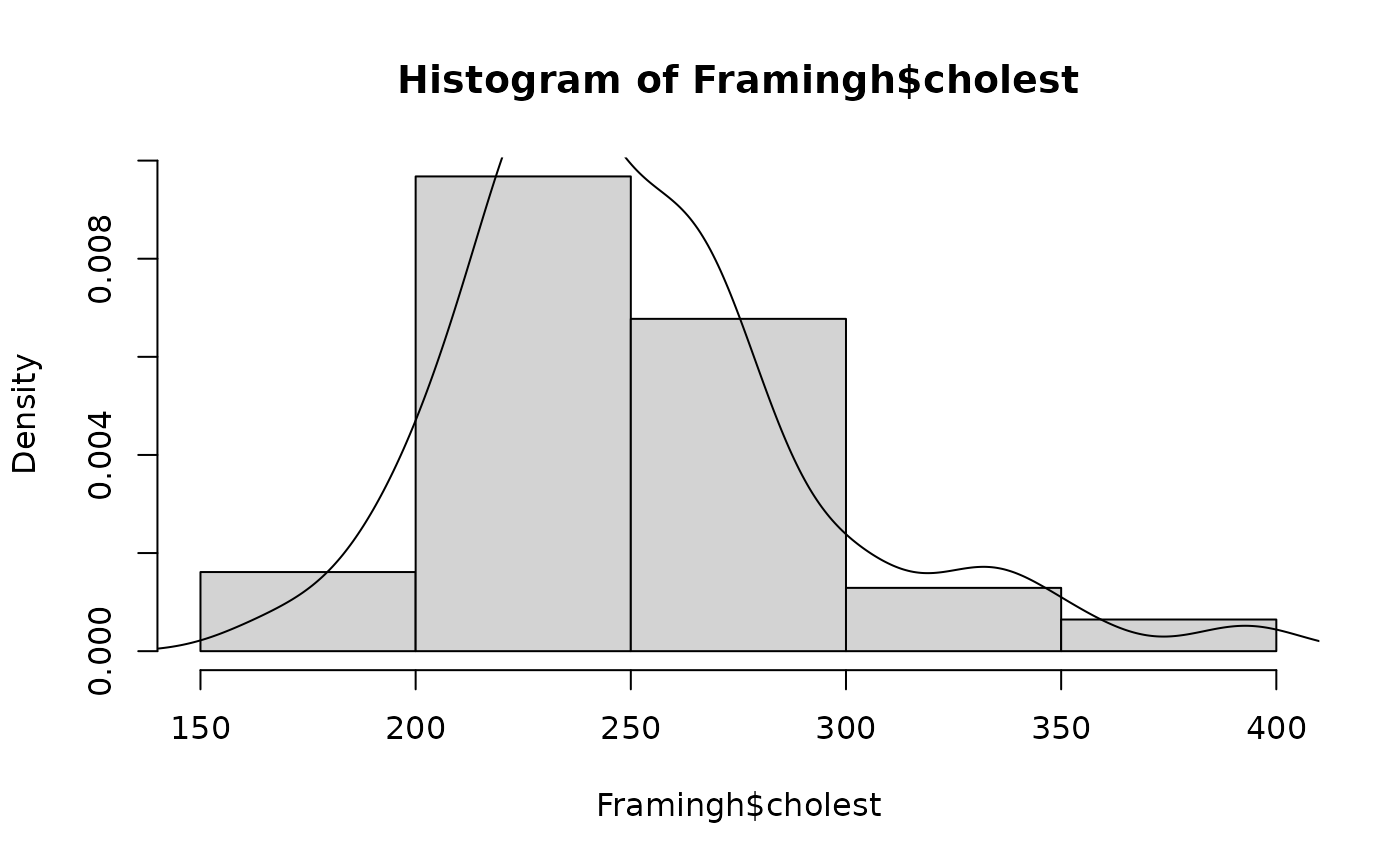Data for Exercises 1.56, 1.75, 3.69, and 5.60

Framingh

## Format

A data frame/tibble with 62 observations on one variable

cholest

a numeric vector with cholesterol values

## Source

R. D'Agostino, et al., (1990) "A Suggestion for Using Powerful and Informative Tests for Normality," The American Statistician, 44 316-321.

## Examples


stem(Framingh$cholest) #> #> The decimal point is 1 digit(s) to the right of the | #> #> 16 | 7 #> 18 | 428 #> 20 | 020125678 #> 22 | 05560000122244668 #> 24 | 03678444668 #> 26 | 34777800288 #> 28 | 35 #> 30 | 008 #> 32 | 746 #> 34 | 3 #> 36 | #> 38 | 3 #> boxplot(Framingh$cholest, horizontal = TRUE)hist(Framingh$cholest, freq = FALSE) lines(density(Framingh$cholest))mean(Framingh$cholest > 200 & Framingh$cholest < 240)
#>  0.4032258

if (FALSE) {
library(ggplot2)
ggplot2::ggplot(data = Framingh, aes(x = factor(1), y = cholest)) +
geom_boxplot() +                 # boxplot
labs(x = "") +                   # no x label
theme_bw() +                     # black and white theme
geom_jitter(width = 0.2) +       # jitter points
coord_flip()                     # Create horizontal plot
ggplot2::ggplot(data = Framingh, aes(x = cholest, y = ..density..)) +
geom_histogram(fill = "pink", binwidth = 15, color = "black") +
geom_density() +
theme_bw()
}